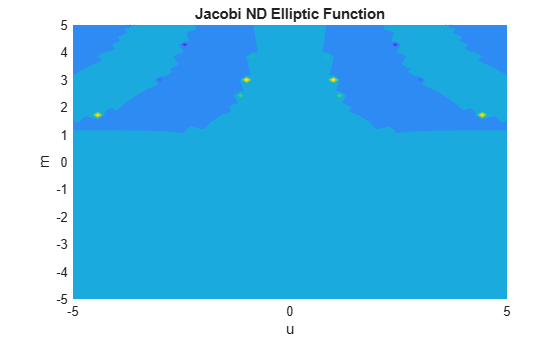# jacobiND

Jacobi ND elliptic function

## Syntax

``jacobiND(u,m)``

## Description

example

````jacobiND(u,m)` returns the Jacobi ND Elliptic Function of `u` and `m`. If `u` or `m` is an array, then `jacobiND` acts element-wise.```

## Examples

collapse all

`jacobiND(2,1)`
```ans = 3.7622```

Call `jacobiND` on array inputs. `jacobiND` acts element-wise when `u` or `m` is an array.

`jacobiND([2 1 -3],[1 2 3])`
```ans = 3.7622 3.2181 -218.7739```

Convert numeric input to symbolic form using `sym`, and find the Jacobi ND elliptic function. For symbolic input where `u = 0` or `m = 0` or `1`, `jacobiND` returns exact symbolic output.

`jacobiND(sym(2),sym(1))`
```ans = cosh(2)```

Show that for other values of `u` or `m`, `jacobiND` returns an unevaluated function call.

`jacobiND(sym(2),sym(3))`
```ans = jacobiND(2, 3)```

For symbolic variables or expressions, `jacobiND` returns the unevaluated function call.

```syms x y f = jacobiND(x,y)```
```f = jacobiND(x, y)```

Substitute values for the variables by using `subs`, and convert values to double by using `double`.

`f = subs(f, [x y], [3 5])`
```f = jacobiND(3, 5)```
`fVal = double(f)`
```fVal = 1.0024```

Calculate `f` to higher precision using `vpa`.

`fVal = vpa(f)`
```fVal = 1.0024338497055006289470589737758```

Plot the Jacobi ND elliptic function using `fcontour`. Set `u` on the x-axis and `m` on the y-axis by using the symbolic function `f` with the variable order `(u,m)`. Fill plot contours by setting `Fill` to `on`.

```syms f(u,m) f(u,m) = jacobiND(u,m); fcontour(f,'Fill','on') title('Jacobi ND Elliptic Function') xlabel('u') ylabel('m')```## Input Arguments

collapse all

Input, specified as a number, vector, matrix, or multidimensional array, or a symbolic number, variable, vector, matrix, multidimensional array, function, or expression.

Input, specified as a number, vector, matrix, or multidimensional array, or a symbolic number, variable, vector, matrix, multidimensional array, function, or expression.

collapse all

### Jacobi ND Elliptic Function

The Jacobi ND elliptic function is

nd(u,m) = 1/dn(u,m)

where dn is the respective Jacobi elliptic function.

The Jacobi elliptic functions are meromorphic and doubly periodic in their first argument with periods 4K(m) and 4iK'(m), where K is the complete elliptic integral of the first kind, implemented as `ellipticK`.

## Version History

Introduced in R2017b